Next: GVF Snake Up: Gradient Vector Flow Field Previous: Gradient Vector Flow (GVF)

## Numerical Implementation

Equations (11a) and (11b) can be solved by treating u and v as functions of time and solving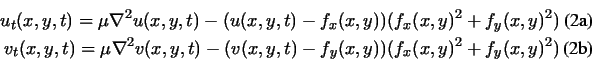The steady-state solution (as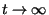) of these linear parabolic equations is the desired solution of the Euler equations (11a) and (11b). Note that these equations are decoupled, and therefore can be solved as separate scalar partial differential equations in u and v. The equations in (12) are known as generalized diffusion equations, and are known to arise in such diverse fields as heat conduction, reactor physics, and fluid flow . For us, they have appeared from our description of desirable properties of external fields for active contours. Diffusion is a natural outcome given the desired filling in'' property.

For convenience, we rewrite Equation (12) as follows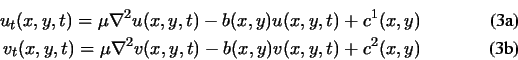where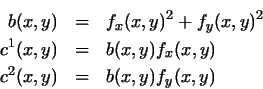Any digital image gradient operator (cf. ) can be used to calculate fx and fy. In the examples shown in this paper, we use simple central differences. The coefficients b(x,y), c1(x,y), and c2(x,y), can then be computed and fixed for the entire iterative process.

To set up the iterative solution, let the indices i, j, and n correspond to x, y, and t, respectively, and let the spacing between pixels be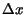and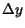and the time step for each iteration be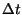. Then the required partial derivatives can be approximated as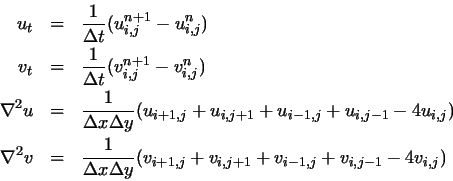Substituting these approximations into (13) gives our iterative solution to GVF: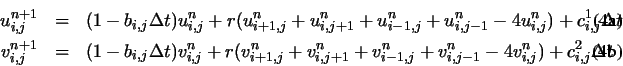where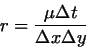(11)

Convergence of the above iterative process is guaranteed by a standard result in the theory of numerical methods (cf. ). Provided that b, c1, and c2 are bounded, (14) is stable whenever the Courant-Friedrichs-Lewy step-size restriction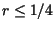is maintained. Since normally,, and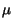are fixed, using the definition of r in (15) we find that the following restriction on the time-stepmust be maintained in order to guarantee convergence of GVF: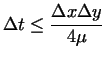(12)

The intuition behind this condition is revealing. First, convergence can be made to be faster on coarser images -- i.e., whenandare larger. Second, whenis large and the GVF is expected to be a smoother field, the convergence rate will be slower (sincemust be kept small).Next: GVF Snake Up: Gradient Vector Flow Field Previous: Gradient Vector Flow (GVF)
Chenyang Xu
1999-11-06